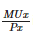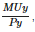Courses

# Test: Theory Of Consumer Behaviour - 5

## 25 Questions MCQ Test Economics Class 12 | Test: Theory Of Consumer Behaviour - 5

Description
This mock test of Test: Theory Of Consumer Behaviour - 5 for Commerce helps you for every Commerce entrance exam. This contains 25 Multiple Choice Questions for Commerce Test: Theory Of Consumer Behaviour - 5 (mcq) to study with solutions a complete question bank. The solved questions answers in this Test: Theory Of Consumer Behaviour - 5 quiz give you a good mix of easy questions and tough questions. Commerce students definitely take this Test: Theory Of Consumer Behaviour - 5 exercise for a better result in the exam. You can find other Test: Theory Of Consumer Behaviour - 5 extra questions, long questions & short questions for Commerce on EduRev as well by searching above.
QUESTION: 1

### The marginal product is above the average product curve, when the average product is:

Solution:

Relationship between AP and MP is stated below:
(i) AP increases when MP is greater than AP.
(ii) AP is maximum when both MP and AP are equal.
(iii) AP decreases when MP is less than AP.
(iv) AP continues to be positive even when MP is zero or negative.

QUESTION: 2

### What does one take on two axes while drawing an indifference curve?

Solution:

An indifference curve is a graph showing combination of two goods(quantity) that give the consumer equal satisfaction and utility. Each point on an indifference curveindicates that a consumer is indifferent between the two and all points give him the same utility.

QUESTION: 3

### A consumer reaches equilibrium when?

Solution:

A consumer is said to be in equilibrium when he feels that he “cannot change his condition either by earning more or by spending more or by changing the quantities of thing he buys”.
A rational consumer will purchase a commodity up to the point where price of the commodity is equal to the marginal utility obtained from the thing.
In equilibrium, the marginal utilities of the different commodities purchased are proportional to their prices and these ratios of marginal utility to price must be equal to the common marginal utility of money.
Hence, MU of X/ Price of X = MU of Y/Price of Y

QUESTION: 4

If the value ofis more than, the consumer__________?

Solution:

MUX/ PX>MUY/PY. In this case, the consumer is getting more marginal utility per rupee in case of good X as compared to Y. Therefore, he will buy more of X and less of Y. This will lead to fall in MUX and rise in MUY. The consumer will continue to buy more of X till MUX/PX = MUY/PY

QUESTION: 5

A demand function shows

Solution:

Demand function shows the relationship between quantity demanded for a particular commodity and the factors influencing it.

QUESTION: 6

The tangency between price line and indifference curve shows?

Solution:

At point of tangency,slope of the both IC and budget line is same,

i.e. MRS=MRE

QUESTION: 7

Consumer surplus is more in the case of______________?

Solution:

Consumer surplus is a measure of the welfare that people gain from consuming goods and servicesConsumer surplus is defined as the difference between the total amount that consumers are willing and able to pay for a good or service (indicated by the demand curve) and the total amount that they actually do pay .

As per Alferd marshall ,CS can not be calculated for luxary goods, inferior goods and necessities.

QUESTION: 8

Marginal utility can be written in terms of symbol as ________?

Solution:
QUESTION: 9

The value of elasticity in case of a downward sloping demand curve will be

Solution:

With a downward-sloping demand curve, price and quantity demanded move in opposite directions, so the price elasticity of demand is always negative. A positive percentage change in price implies a negative percentage change in quantity demanded, and vice versa.

QUESTION: 10

The value of elasticity in case of a rectangular hyperbola demand curve will be

Solution:

Rectangular hyperbola curve said to be unitary elastic as elasticity along the demand curve is same.

QUESTION: 11

A consumer reaches equilibrium at the point where:

Solution:

To determine the equilibrium point, consumer compares the price (or cost) of the given commodity with its utility (satisfaction or benefit). Being a rational consumer, he will be at equilibrium when marginal utility is equal to price paid for the commodity.

QUESTION: 12

If price elasticity of demand for a good is (-) 3.If the price rises from Rs. 10 to Rs. 12 per unit, what is the percentage change in demand?

Solution:

ed=ed= 3

% change in quantity demanded =?

% change in price = (12−10/10)∗100=20(12−10/10)∗100=20%

by using %age method to calculate elasticity

60%

QUESTION: 13

If price elasticity of demand for a good is (-) 2.If the price falls from Rs. 8 the consumer buys 50 percent more, what is the new price?

Solution:

ed = % change in quantity demanded / % change in price

% change in quantity demanded [= 50

ed = 2

% change in price = X

by using above formula

50 / X = 2

X = 25

25% of 8 is 2.

new price 8-2 = 6.

QUESTION: 14

Due to a 10 percent fall in the price of a good, its demand rises from 400 units to 450 units. Calculate its price elasticity of demand.

Solution:

Price elasticity of demand is a measure of the change in the quantity demanded or purchased of a product in relation to its price change.
Price Elasticity of Demand = % Change in Quantity Demanded / % Change in Price
% Change in Quantity = 50/400 x 100 = 12.5
% Change in Price = 10
12.5/10 = 1.25

QUESTION: 15

Due to a rise in the price of a good by Rs.5, its demand rises from 100 units to 95 units. Its price elasticity of demand is (-) 1.2. Calculate the price before the change.

Solution:

ed = (ΔQ/ ΔP) * (P/Q)

ΔQ = 5

ΔP = 5

P = ?

Q= 100

ed = 1.2

by using above formula

1.2 = (5/5)*(P/100)

P = 120

QUESTION: 16

The elasticity of demand on different points on a linear demand curve is different. It is

Solution:

As per geomeric method for calculating price elasticity,we can say it can be different.

slope remains constant as it is linear curve but not the elsaticity, it can be different.

QUESTION: 17

What does monotonicity of preferences imply?

Solution:

An agent's preferences are said to be stronglymonotonic if, given a consumption bundle , the agent prefers all consumption bundles that have more of at least one good, and not less in any other good.

QUESTION: 18

What will you say about MU when TU is maximum?

Solution:

MU & TU relationship  ;

MU is the rate of change of TU.

When the MU decreases, TU increases at decreasing rate.

When MU becomes zero, TU is maximum. It is a saturation point.

When MU becomes negative, TU declines

QUESTION: 19

Suppose a consumer’s preferences are monotonic. What can you say about his preference ranking over the bundles (10,10),(10,9) and (9,9)?

Solution:

A consumer's preferences are said to be stronglymonotonic if, given a consumption bundle , the consumer prefers all consumption bundles that have more of at least one good, and not less in any other good.

QUESTION: 20

Suppose the price elasticity of demand for a good is – 0.2. How will the expenditure on the good be affected if there is a 10 % increase in the price of the good?

Solution:

As per total outlay method

(i) If with a fall in price (demand increases) the total expenditure increases or with a rise in price (demand falls), the total expenditure falls, in that case the elasticity of demand is greater than one i.e. ED > 1.

(ii) If with a rise or fall in the price (demand falls or rises respectively), the total expenditure remains the same, the demand will be unitary elastic or ED = 1.

(iii) If with a fall in price (Demand rises), the total expenditure also falls, and with a rise in price (Demand falls) the total expenditure also rises, the demand is said to be less classic or elasticity of demand is less than one (ED < 1).

QUESTION: 21

A consumer buys 50 units of a good at Rs. 4/- per unit. When its price falls by 25 percent its demand rises to 100 units then the price elasticity of demand will be

Solution:

ed = % change in quantity demanded / % change in price

% change in quantity = (100-50) / 50 ) * 100 = 100%

% change in price = 25%

by using above formula ;

100/25 = 4

QUESTION: 22

Price elasticity of demand for wheat is equal to unity and a household demands 40 Kg of wheat when the price is Rs.1 per kg. At what price will the household demand 20 kg of wheat?

Solution:

If elasticity of demand is equal to 1.

then,

% change in price = % change in quantity

there is 50% fall in quantity from 40 to 20 kg.

hence there should be 50% increase in price

new price = 1.5

QUESTION: 23

Which of the following law states that the more a consumer consumes of a product the less is the utility he derives from the additional consumption?

Solution:

Other things remaining the same when a person takes successive units of a commodity, the marginal utility diminishes constantly

QUESTION: 24

In case of relatively more elastic, demand curve is:

Solution:

A relatively elastic demand curve has a steeper slope.A relatively elastic demand has a flatter curve because the percentage change in quantity demanded is greater than a percentage change in price.

QUESTION: 25

How would you calculate the new partner’s capital, when it is not given in the question?

Solution:

Calculation of new partner’s capital should be done as follows:
1.Calculated the combined or adjusted capitals of all the existing partners (after all adjustments)
2.Find out the reciprocal of remaining share
3.Now, combined capitals x reciprocal of remaining share x new partner’s share